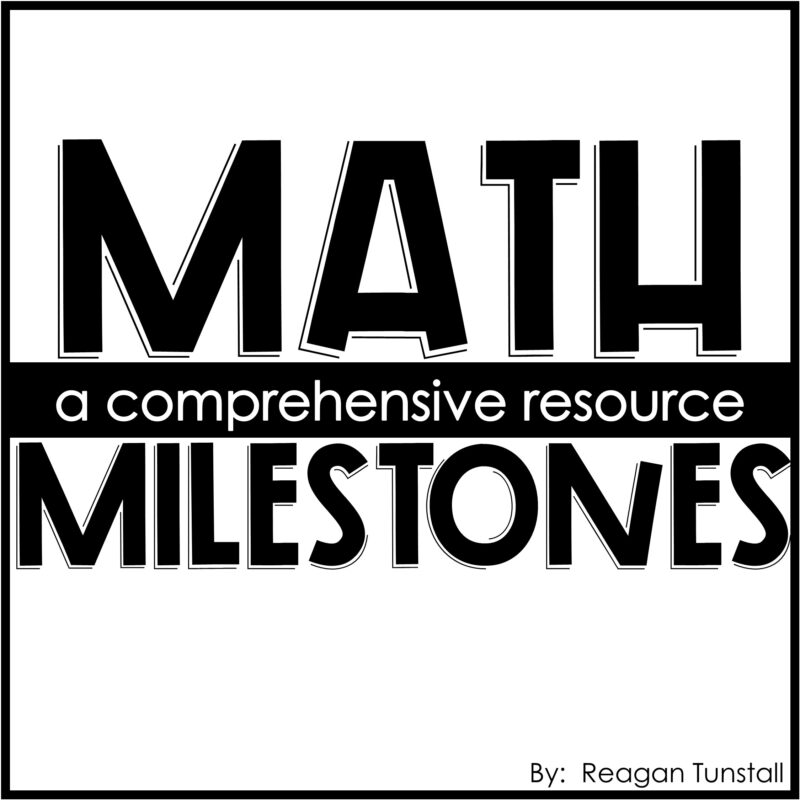# Building Number Sense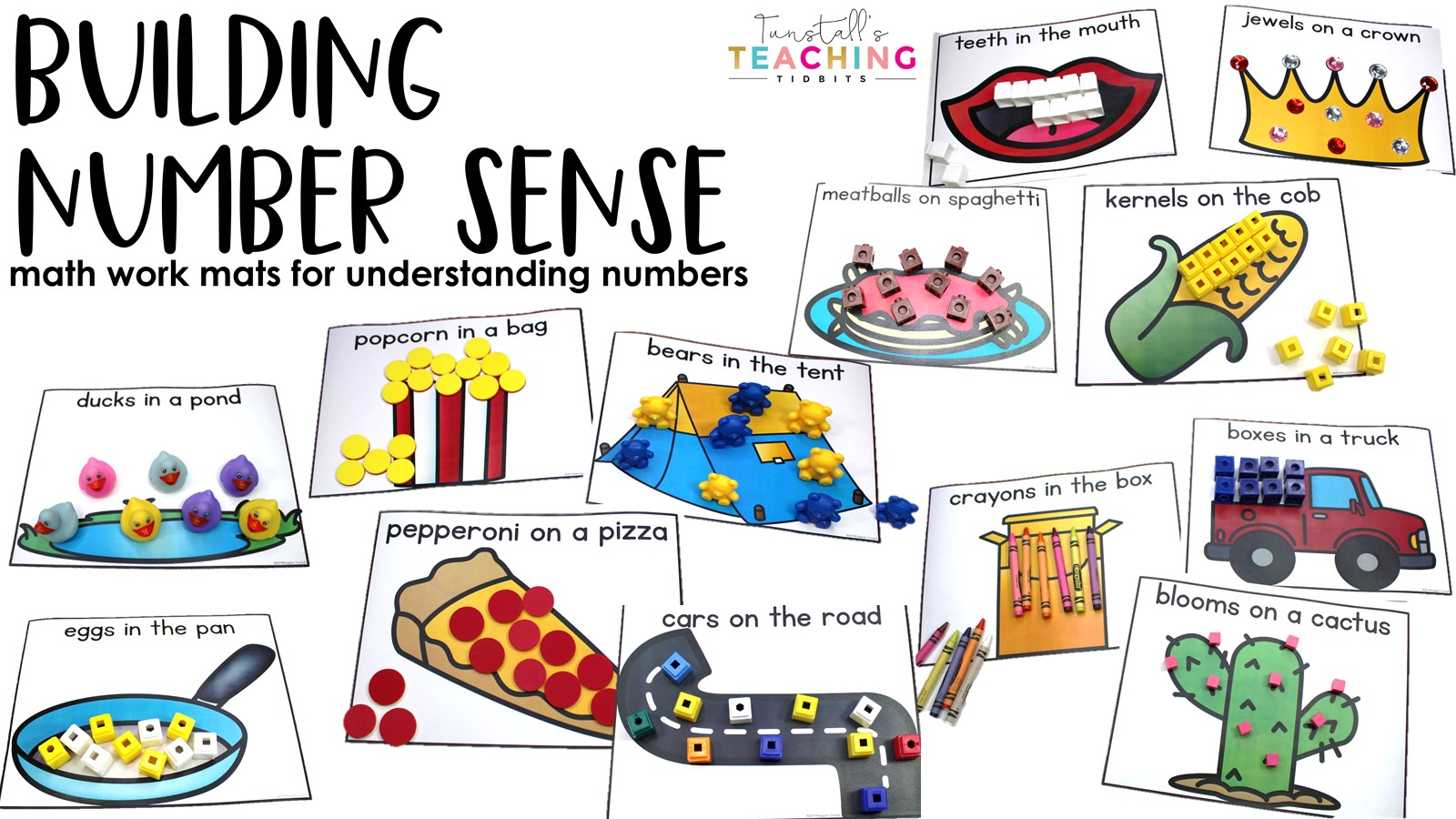A strong foundation in number sense is an important math milestone.   When students count sets, order numbers, group objects, and compare values, they pave their way to computation.  In this post, we will learn a simple trick to help students with building number sense.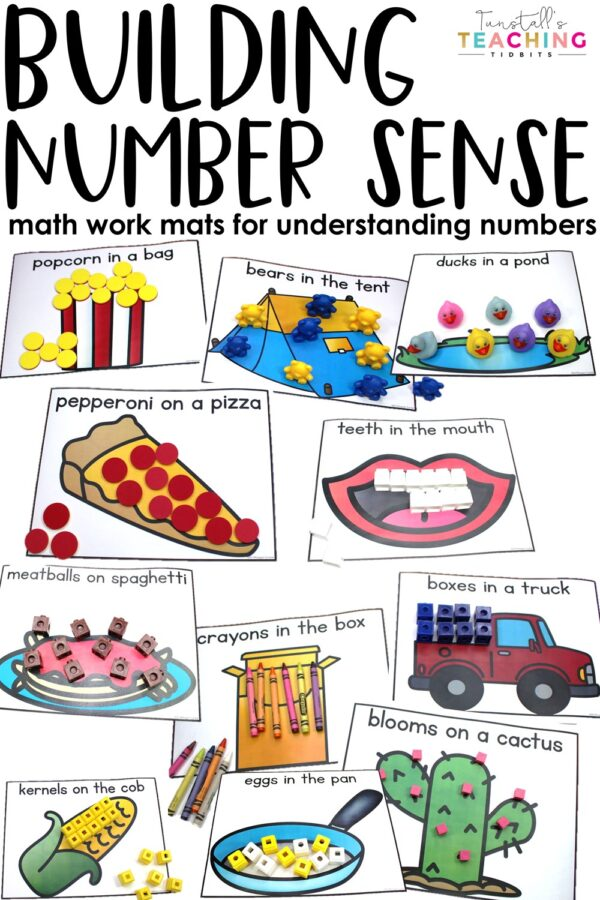## BUILDING NUMBER SENSE

Learning to count, identify numbers, and understand quantity are a few of the essential concepts of building number sense.  When students are ages 3-5 they are in a stage of mathematical development centered around understanding representation.  By using simple number sense picture mats, we engage our brains in the world of math with numbers.  In fact, a simple manipulative like the snap cube is transformed into knights in a castle or meatballs on a pile of spaghetti.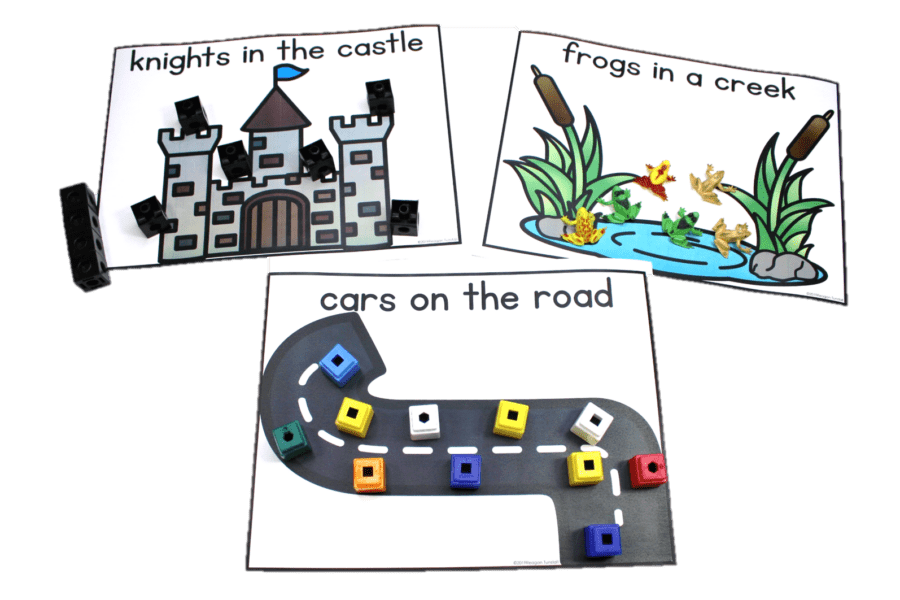Children naturally access their imaginations in day-to-day life.  We take advantage of student creativity when we purposefully create the scene for math learning. For example, playing dentist easily becomes a subtraction lesson.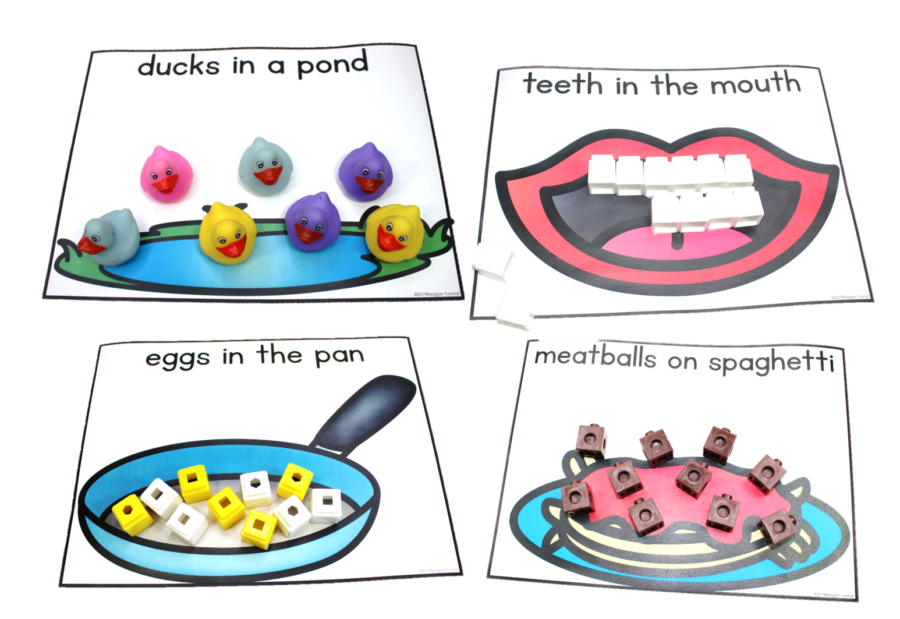So, what do we do with the building number sense mats?  Here’s a list of skills that students need within this age and range of math milestone.

### Representing

• match touch to counting (one-to-one)
• simply classifying color, shape, texture
• ordering (by height by size or desire of mathematician)
• sequencing (desire present to create order in some way)
• count to 10, then to 25
• recognize circle, square, triangle, rectangle
• recognize numbers to 10/25 flashcards
• visual clustering
• subitizing
• learning to match sets of objects by number
• recognize penny, nickel, dime, quarter
• positional words (put the penny in front of the pencil)
• which is different? (numbers & shapes)
• which are the same as? (numbers & shapes)
• space organization/spatial orientation
• pattern recognition
• pattern extension
• estimation
• deductive thinking
• create a pattern or number with one or two objects

## MATH STRATEGIES

While these representing skills are at the forefront, we teach students the following math strategies.

• build it
• five frame
• ten frame
• dots
• make Xs
• cross out
• draw it
• quick sketch
• make it
• number line to 10
• count on
• fingers
• counters
• model it
• number chart
• patterns
• act it out
• number sentence
• make a set
• estimate
• order

The added layer of teaching strategies for our skills creates empowered mathematicians.  I teach the strategy and we immediately apply it in our math learning.  More These visuals help anchor the learning for students.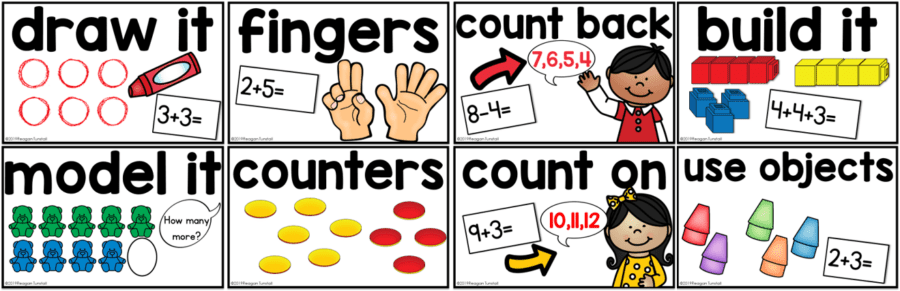## MORE ON MATH MILESTONES

Visit this blog post to follow the math milestones from 0-12 years of age.  Grab the free download to keep in your guided math binder or lesson plans for reference!